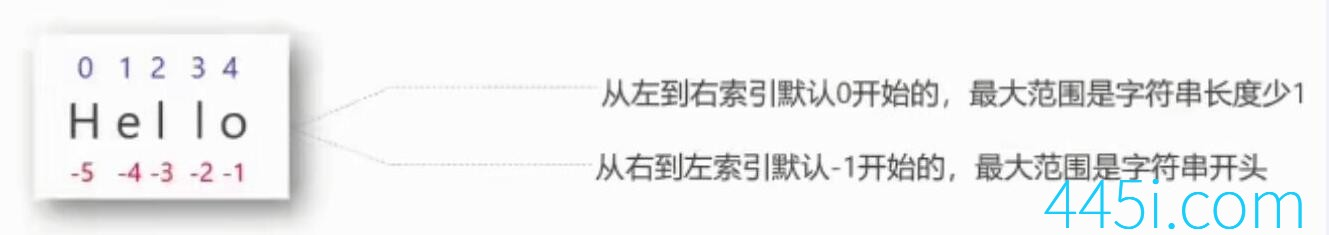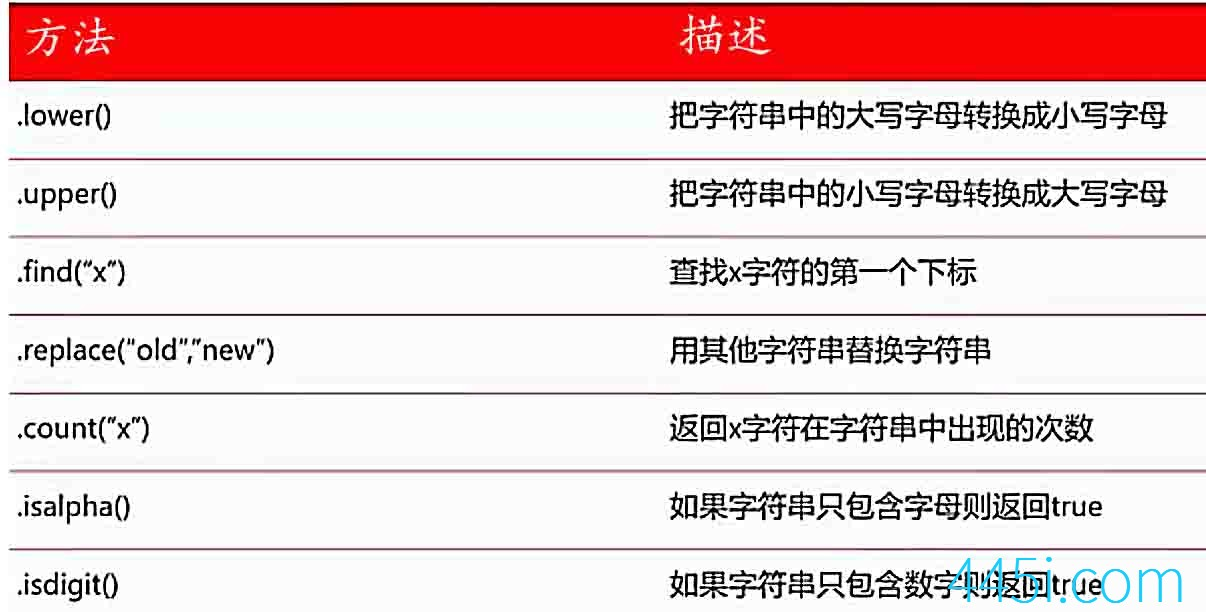# Python零基础入门教程6:字符串

`url = 'www.445i.com'print(url)`

```url = 'www.445i.com'

print(url)
```

`url = 'www.445i.com'print(url[-1])````word = 'Hello'
print(word)```

`word = 'Hello'print(len(word))`

```word = '我来了啊'
print(len(word))```

`word = '我来 了啊'print(len(word))``word = 'Hello'print(word.lower())`

```word = 'Hello'
print(word.upper())```

`print('你好，' + '可以交个朋友吗？')`

`i = '232'w = '123.0'print(int(i),float(w))print(type(i),type(w))`

``````232 123.0
<class 'str'> <class 'str'>``````

### 觉得文章有用就打赏一下文章作者

#### 支付宝扫一扫打赏#### 微信扫一扫打赏